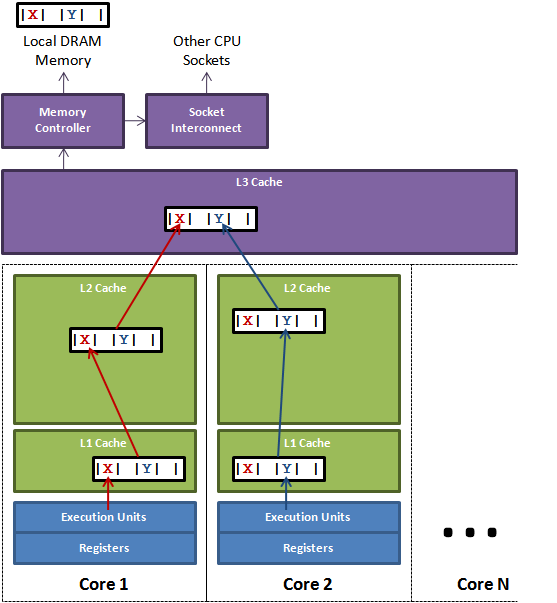false-sharing

# 伪共享和内存可见性(False Sharing 和 Memory Visibility)

## 伪共享False Sharing

Cache Line 现在X86-64 一般是64个字节一行。我们可以对变量进行补齐操作，以此来避免伪共享。

    static class BadPopularObject {
public volatile long usefulVal;

}

static class GoodPopularObject {
public volatile long usefulVal;
public volatile long t1, t2, t3, t4, t5, t6, t7;
}


    static class GoodPopularObject {
public volatile long usefulVal;
public volatile long t1, t2, t3, t4, t5, t6, t7;

public long preventOptimization() {
return t1 + t2 + t3 + t4 + t5 + t6 + t7;
}
}


    static class SomePopularObject {
@sun.misc.Contended
public volatile long usefulVal;
public volatile long anotherVal;
}


static class PaddingAtomicLong extends AtomicLong {
public volatile long t1, t2, t3, t4, t5, t6, t7;

public long preventOptimization() {
return t1 + t2 + t3 + t4 + t5 + t6 + t7;
}
}

static class FSTest implements Runnable {
public final static int NUM_THREADS = 4;
public final static long ITERATIONS = 100L * 1000L * 1000L;
public static AtomicLong[] longs = new AtomicLong[NUM_THREADS];
public final int index;
static {
for (int i = 0; i < NUM_THREADS; i++) {
longs[i] = new AtomicLong();
}
}

public FSTest(int index) {
this.index = index;
}

public void run() {
long i = ITERATIONS + 1;
while (i-- > 0) {
longs[index].set(i);
}
}

public static void runTest() {
for (int i = 0; i < FSTest.NUM_THREADS; i++) {
FSTest fs = new FSTest(i);
ts[i].start();
}
for (Thread t : ts) {
try {
t.join();
} catch (InterruptedException e) {
// TODO Auto-generated catch block
e.printStackTrace();
}
}
}

}

static class NFSTest implements Runnable {
public final static int NUM_THREADS = 4;
public final static long ITERATIONS = 100L * 1000L * 1000L;
public final int index;
static {
for (int i = 0; i < NUM_THREADS; i++) {
}
}

public NFSTest(int index) {
this.index = index;
}

public void run() {
long i = ITERATIONS + 1;
while (i-- > 0) {
longs[index].set(i);
}
}

public static void runTest() {
for (int i = 0; i < NFSTest.NUM_THREADS; i++) {
NFSTest nfs = new NFSTest(i);
ts[i].start();
}
for (Thread t : ts) {
try {
t.join();
} catch (InterruptedException e) {
// TODO Auto-generated catch block
e.printStackTrace();
}
}
}

}

public static void main(String[] args) {
long start = System.nanoTime();
FSTest.runTest();
System.out.println(System.nanoTime() - start);

start = System.nanoTime();
NFSTest.runTest();
System.out.println(System.nanoTime() - start);
}

\\8287659690
\\939645571